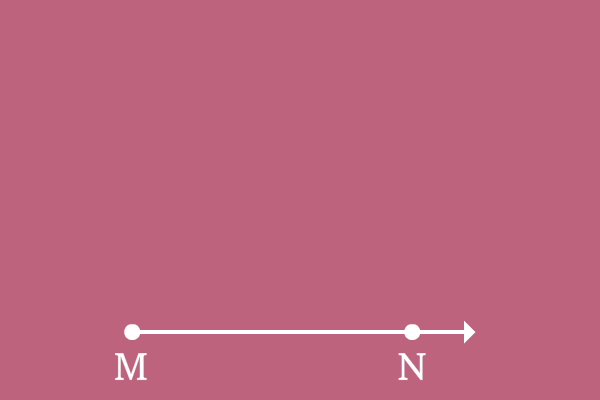# Positive angle

## Definition

The amount of rotation of a ray from its initial position to final position in anticlockwise direction is called positive angle.

Anticlockwise direction is considered as positive direction in the case of angle. There is a universally accepted reason to consider anticlockwise direction as positive direction. Every book is opened in anticlockwise direction and we feel positively to start reading or writing. So, the anticlockwise direction is considered as positive direction in the case of angle.

Positive angles are written by writing with or without plus sign $(+)$ before the angle.

### Example$\overrightarrow{MN}$ is a ray and it is initially at a position on the plane.

The ray $\overrightarrow{MN}$ is rotated in anticlockwise direction and reached its final position where it is known as ray $\overrightarrow{MO}$.

The direction of angle made by the ray is anticlockwise direction and it is considered as positive direction.

Hence, the angle made by the ray $\overrightarrow{MN}$ to become $\overrightarrow{MO}$ is known as positive angle.

For example, if angle is $30^°$ and it is made in anticlockwise direction, it is written as $30^°$ or $+30^°$ in mathematics.

Latest Math Topics
Jun 26, 2023
Jun 23, 2023

Latest Math Problems
Jul 01, 2023
Jun 25, 2023
###### Math Questions

The math problems with solutions to learn how to solve a problem.

Learn solutions

Practice now

###### Math Videos

The math videos tutorials with visual graphics to learn every concept.

Watch now

###### Subscribe us

Get the latest math updates from the Math Doubts by subscribing us.